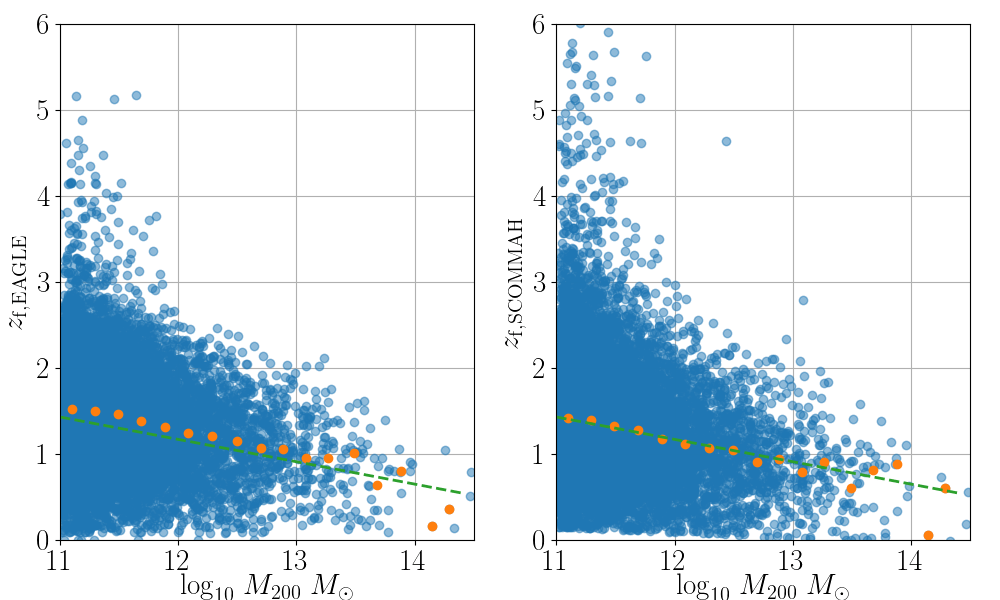# SCOMMAH

Scommah stands for the Scatter in the COncentration Mass relation and halo Mass Accretion History. It is a python module that follows the semianalytic formalism of Correa et al. (2020, in prep.) to calculate a halo’s formation time given its virial mass and concentration parameter.

The module uses routines in numpy and scipy to create a structured dataset with mass accretion rates, redshifts and halo formation times. It assumes the halo virial mass, M200 (defined to be all mass enclosed within the halo virial radius, R200, where the mean density is 200 times the critical density) in units of log10[Msun], and the concentration, c200, is defined as the ratio of the halo virial radius over scale radius (obtained from best-fit NFW profile).

Scommah returns the redshift of halo formation, zf, defined as the redshift at which the halo mass is half its z=0 value, M(z=zf)=M(z=0)/2, as well as the halo mass history.Example of the formation time-halo mass relation from EAGLE haloes (left) and the relation predicted by SCOMMAH (right).

### Getting started

You can begin by getting the code

``git clone [email protected]:correac/scommah.git``

Test scommah by running `example.py`, which uses scommah to determine the formation redshift and mass accretion history of a halo given the user input.

``````cd scommah/scommah
\$ python example.py
``````

For this example scommah requires as input z=0 haloes’ mass (M200) and concentrations (c200).

Alternatively you can run scommah as follows

``````\$ cd scommah/scommah
\$ python
>> from scommah import scommah_zf
>> zf = scommah_zf(7.8,12.1)    #Outputs redshift of formation of 10^12.1Msun halo with c200=7.8
>> from scommah import scommah_Mz
>> Mz = scommah_Mz(zf,7.8,12.1) #Outputs halo mass as a function of redshift in dictonary form: {'Mz':Mz, 'z': z}
``````

Additionally you can run `example_with_eagle_data.py` and `example_with_clash_data.py`. The first example tests how scommah performs in comparison with the outputs from the EAGLE dark matter only simulation. Note that to run this example you need to first download the EAGLE database (1.3G) here. Unzip the data_mahstery folder. `example_with_eagle_data.py` will ask you to specify the path to the folder. Run this example as

``````\$ cd scommah/scommah
\$ python example_with_eagle_data.py
``````

The example `example_with_clash_data.py` can be directly run as follows

``````\$ cd scommah/scommah
\$ python example_with_clash_data.py
``````

and ouputs two plots in png format, the c200-M200 relation for the data as well as the zformation-M200 relation, and two txt files containing the mass, concentration and formation time calculated by scommah.